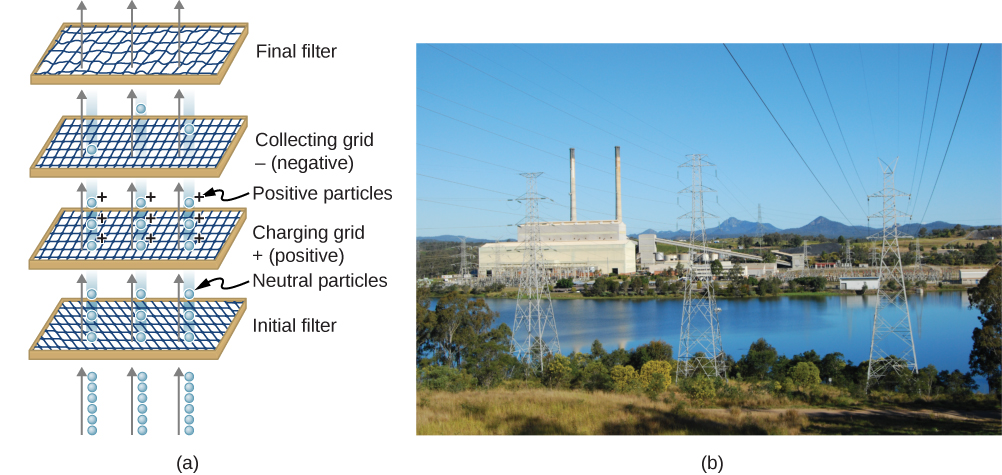# 7.6 Applications of electrostatics  (Page 3/12)

 Page 3 / 12

Large electrostatic precipitators    are used industrially to remove over $99%$ of the particles from stack gas emissions associated with the burning of coal and oil. Home precipitators, often in conjunction with the home heating and air conditioning system, are very effective in removing polluting particles, irritants, and allergens.(a) Schematic of an electrostatic precipitator. Air is passed through grids of opposite charge. The first grid charges airborne particles, while the second attracts and collects them. (b) The dramatic effect of electrostatic precipitators is seen by the absence of smoke from this power plant. (credit b: modification of work by “Cmdalgleish”/Wikimedia Commons)

## Summary

• Electrostatics is the study of electric fields in static equilibrium.
• In addition to research using equipment such as a Van de Graaff generator, many practical applications of electrostatics exist, including photocopiers, laser printers, ink jet printers, and electrostatic air filters.

## Key equations

 Potential energy of a two-charge system $U\left(r\right)=k\frac{qQ}{r}$ Work done to assemble a system of charges ${W}_{12\cdots N}=\frac{k}{2}\sum _{i}^{N}\sum _{j}^{N}\frac{{q}_{i}{q}_{j}}{{r}_{ij}}\phantom{\rule{0.2em}{0ex}}\text{for}\phantom{\rule{0.2em}{0ex}}i\ne j$ Potential difference $\text{Δ}V=\frac{\text{Δ}U}{q}\phantom{\rule{0.2em}{0ex}}\text{or}\phantom{\rule{0.2em}{0ex}}\text{Δ}U=q\text{Δ}V$ Electric potential $V=\frac{U}{q}=-{\int }_{R}^{P}\stackrel{\to }{E}\cdot d\stackrel{\to }{l}$ Potential difference between two points $\text{Δ}{V}_{AB}={V}_{B}-{V}_{A}=\text{−}{\int }_{A}^{B}\stackrel{\to }{\text{E}}·d\stackrel{\to }{\text{l}}$ Electric potential of a point charge $V=\frac{kq}{r}$ Electric potential of a system of point charges ${V}_{P}=k\sum _{1}^{N}\frac{{q}_{i}}{{r}_{i}}$ Electric dipole moment $\stackrel{\to }{\text{p}}=q\stackrel{\to }{\text{d}}$ Electric potential due to a dipole ${V}_{P}=k\frac{\stackrel{\to }{\text{p}}·\stackrel{^}{\text{r}}}{{r}^{2}}$ Electric potential of a continuous charge distribution ${V}_{P}=k\int \frac{dq}{r}$ Electric field components ${E}_{x}=-\frac{\partial V}{\partial x},\phantom{\rule{0.2em}{0ex}}{E}_{y}=-\frac{\partial V}{\partial y},\phantom{\rule{0.2em}{0ex}}{E}_{z}=-\frac{\partial V}{\partial z}$ Del operator in Cartesian coordinates $\stackrel{\to }{\nabla }=\stackrel{^}{\text{i}}\frac{\partial }{\partial x}+\stackrel{^}{\text{j}}\frac{\partial }{\partial y}+\stackrel{^}{\text{k}}\frac{\partial }{\partial z}$ Electric field as gradient of potential $\stackrel{\to }{\text{E}}=\text{−}\stackrel{\to }{\nabla }V$ Del operator in cylindrical coordinates $\stackrel{\to }{\nabla }=\stackrel{^}{\text{r}}\frac{\partial }{\partial r}+\stackrel{^}{\mathit{\text{φ}}}\frac{1}{r}\phantom{\rule{0.2em}{0ex}}\frac{\partial }{\partial \phi }+\stackrel{^}{\text{z}}\frac{\partial }{\partial z}$ Del operator in spherical coordinates $\stackrel{\to }{\nabla }=\stackrel{^}{\text{r}}\frac{\partial }{\partial r}+\stackrel{^}{\mathit{\text{θ}}}\frac{1}{r}\phantom{\rule{0.2em}{0ex}}\frac{\partial }{\partial \theta }+\stackrel{^}{\mathit{\text{φ}}}\frac{1}{r\phantom{\rule{0.2em}{0ex}}\text{sin}\phantom{\rule{0.2em}{0ex}}\theta }\phantom{\rule{0.2em}{0ex}}\frac{\partial }{\partial \phi }$

## Conceptual questions

Why are the metal support rods for satellite network dishes generally grounded?

So that lightning striking them goes into the ground instead of the television equipment.

(a) Why are fish reasonably safe in an electrical storm? (b) Why are swimmers nonetheless ordered to get out of the water in the same circumstance?

What are the similarities and differences between the processes in a photocopier and an electrostatic precipitator?

They both make use of static electricity to stick small particles to another surface. However, the precipitator has to charge a wide variety of particles, and is not designed to make sure they land in a particular place.

About what magnitude of potential is used to charge the drum of a photocopy machine? A web search for “xerography” may be of use.

## Problems

(a) What is the electric field 5.00 m from the center of the terminal of a Van de Graaff with a 3.00-mC charge, noting that the field is equivalent to that of a point charge at the center of the terminal? (b) At this distance, what force does the field exert on a $2.00\text{-}\mu \text{C}$ charge on the Van de Graaff’s belt?

(a) What is the direction and magnitude of an electric field that supports the weight of a free electron near the surface of Earth? (b) Discuss what the small value for this field implies regarding the relative strength of the gravitational and electrostatic forces.

a. $F=5.58\phantom{\rule{0.2em}{0ex}}×\phantom{\rule{0.2em}{0ex}}{10}^{-11}\phantom{\rule{0.2em}{0ex}}\text{N/C}$ ;

The electric field is towards the surface of Earth. b. The coulomb force is much stronger than gravity.

Maxwell's stress tensor is
if 6.0×10^13 electrons are placed on a metal sphere of charge 9.0micro Coulombs, what is the net charge on the sphere
18.51micro Coulombs
ASHOK
Is it possible to find the magnetic field of a circular loop at the centre by using ampere's law?
Is it possible to find the magnetic field of a circular loop at it's centre?
yes
Brother
The density of a gas of relative molecular mass 28 at a certain temperature is 0.90 K kgmcube.The root mean square speed of the gas molecules at that temperature is 602ms.Assuming that the rate of diffusion of a gas in inversely proportional to the square root of its density,calculate the density of
A hot liquid at 80degree Celsius is added to 600g of the same liquid originally at 10 degree Celsius. when the mixture reaches 30 degree Celsius, what will be the total mass of the liquid?
what is electrostatics
Study of charges which are at rest
himanshu
Explain Kinematics
Two equal positive charges are repelling each other. The force on the charge on the left is 3.0 Newtons. Using your notes on Coulomb's law, and the forces acting on each of the charges, what is the force on the charge on the right?
Using the same two positive charges, the left positive charge is increased so that its charge is 4 times LARGER than the charge on the right. Using your notes on Coulomb's law and changes to the charge, once the charge is increased, what is the new force of repulsion between the two positive charges?
Nya
A mass 'm' is attached to a spring oscillates every 5 second. If the mass is increased by a 5 kg, the period increases by 3 second. Find its initial mass 'm'
a hot water tank containing 50,000g of water is heated by an electric immersion heater rated at 3kilowatt,240volt, calculate the current
what is charge
product of current and time
Jaffar
Why always amber gain electrons and fur loose electrons? Why the opposite doesn't happen?
A closely wound search coil has an area of 4cm^2,1000 turns and a resistance of 40ohm. It is connected to a ballistic galvanometer whose resistance is 24 ohm. When coil is rotated from a position parallel to uniform magnetic field to one perpendicular to field,the galvanometer indicates a charge
Using Kirchhoff's rules, when choosing your loops, can you choose a loop that doesn't have a voltage?
how was the check your understand 12.7 solved?By John GabrieliBy OpenStaxBy OpenStaxBy OpenStaxBy Saylor FoundationBy Janet ForresterBy Carly AllenBy RhodesBy John GabrieliBy Sandhills MLT